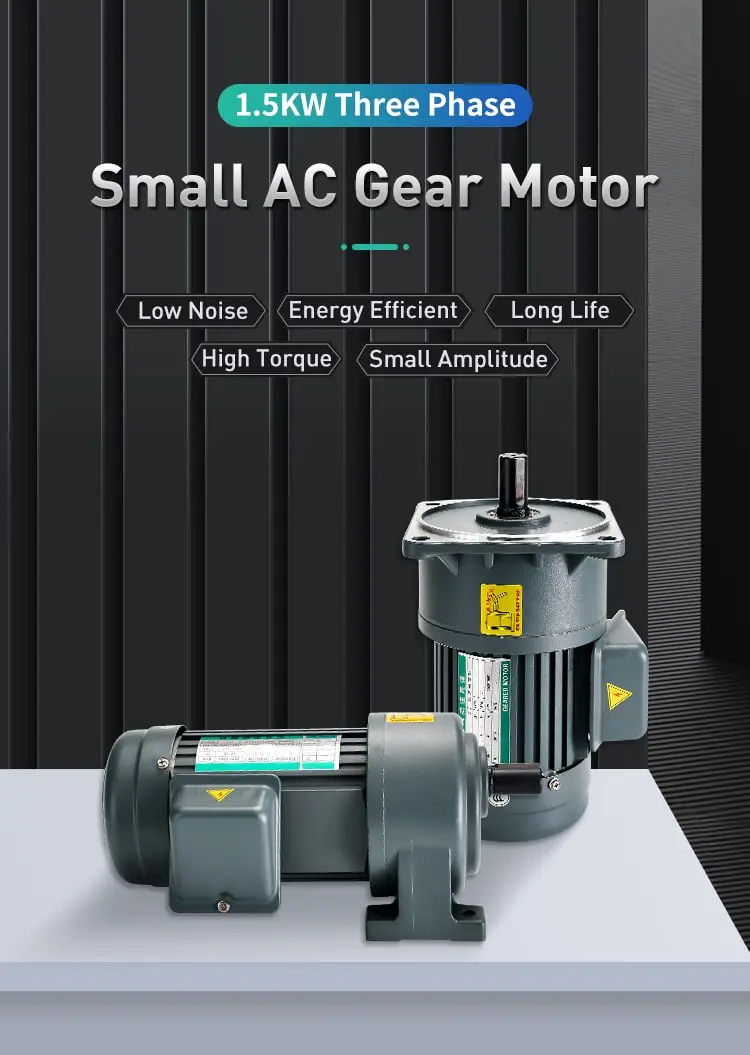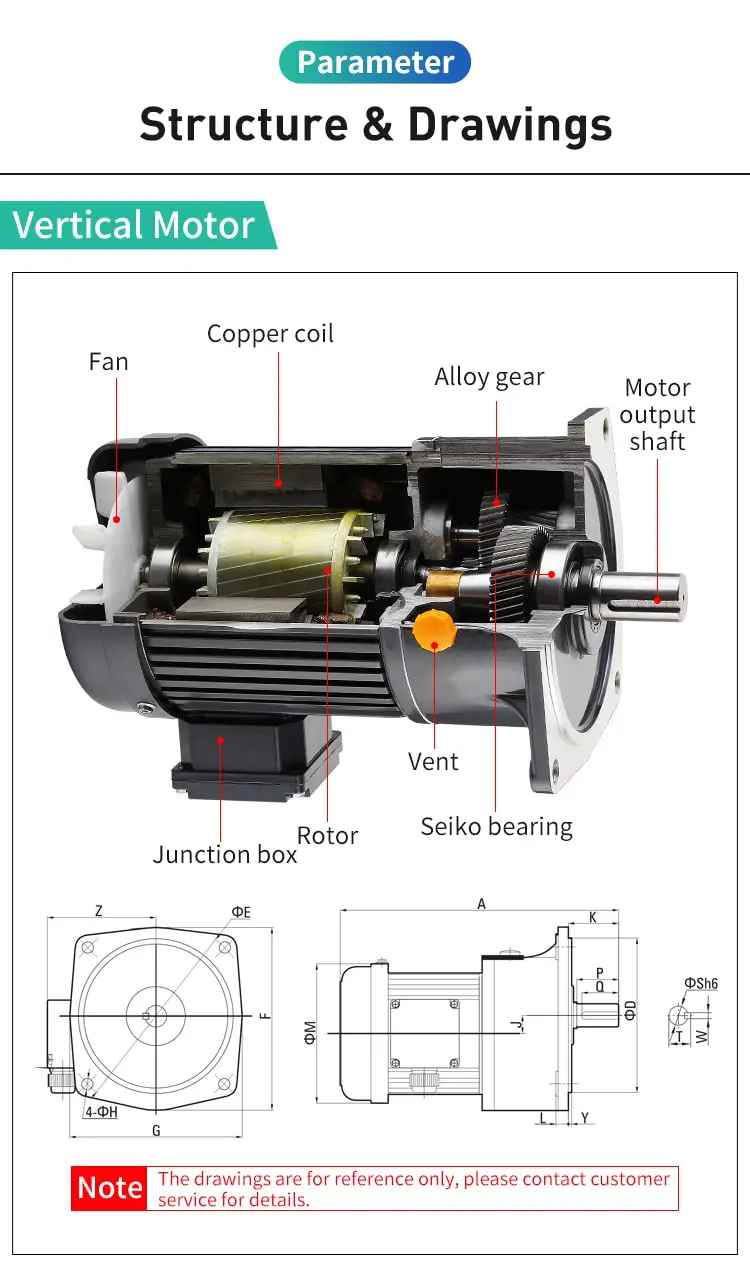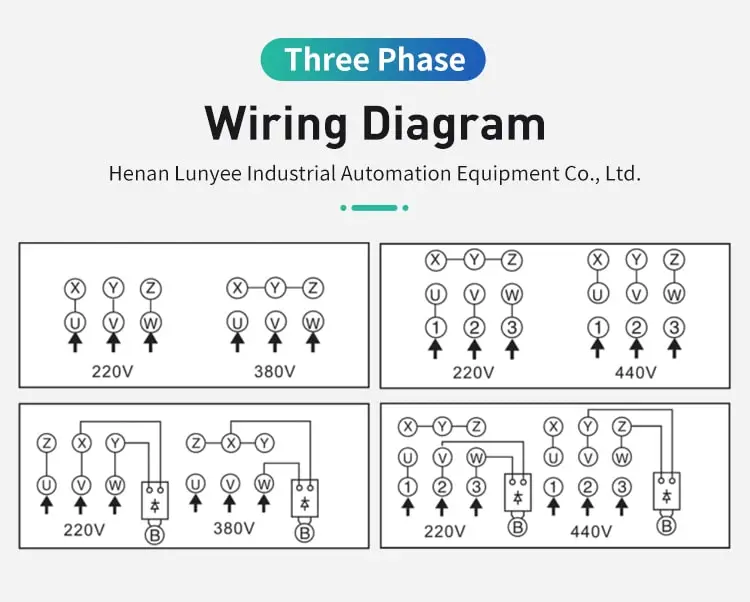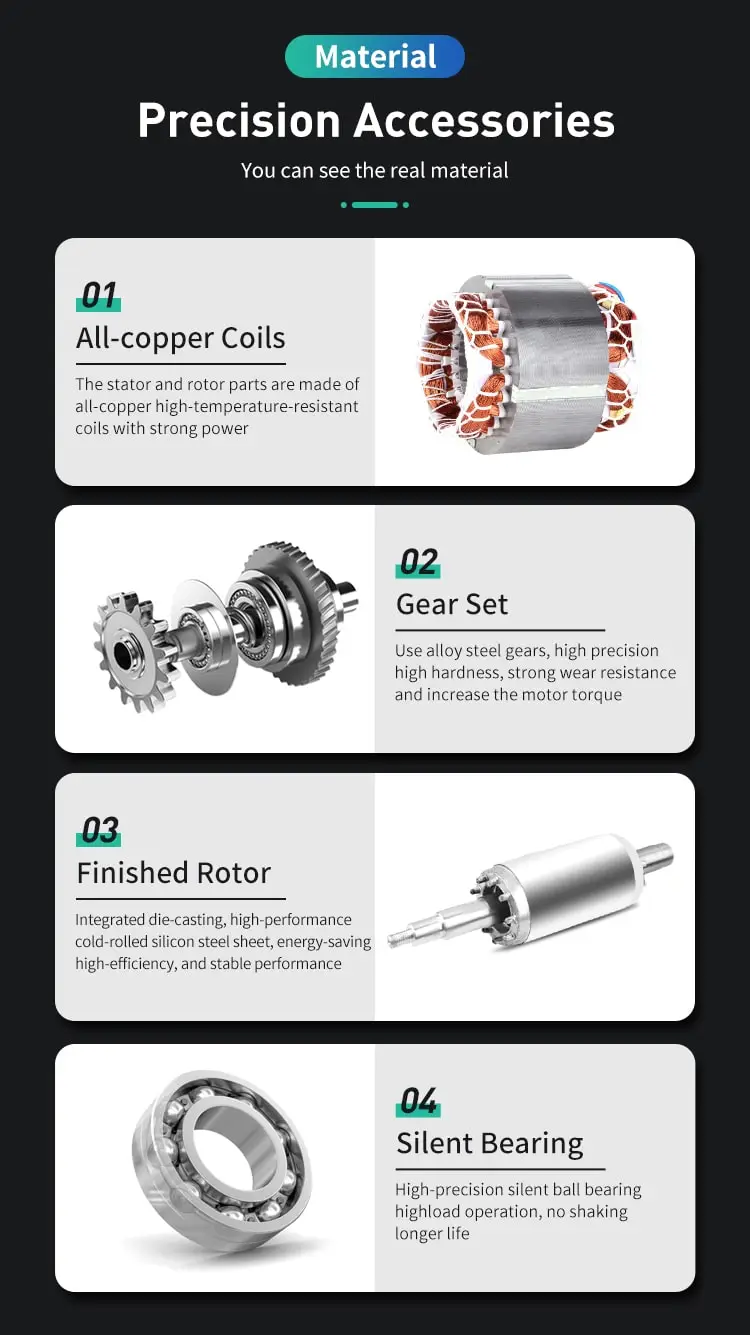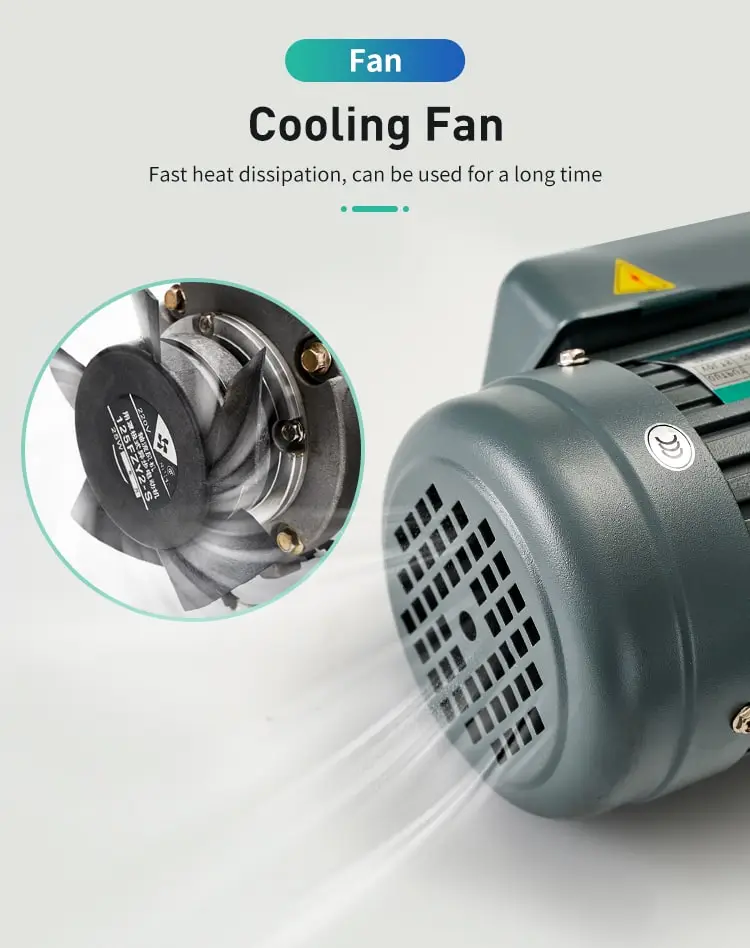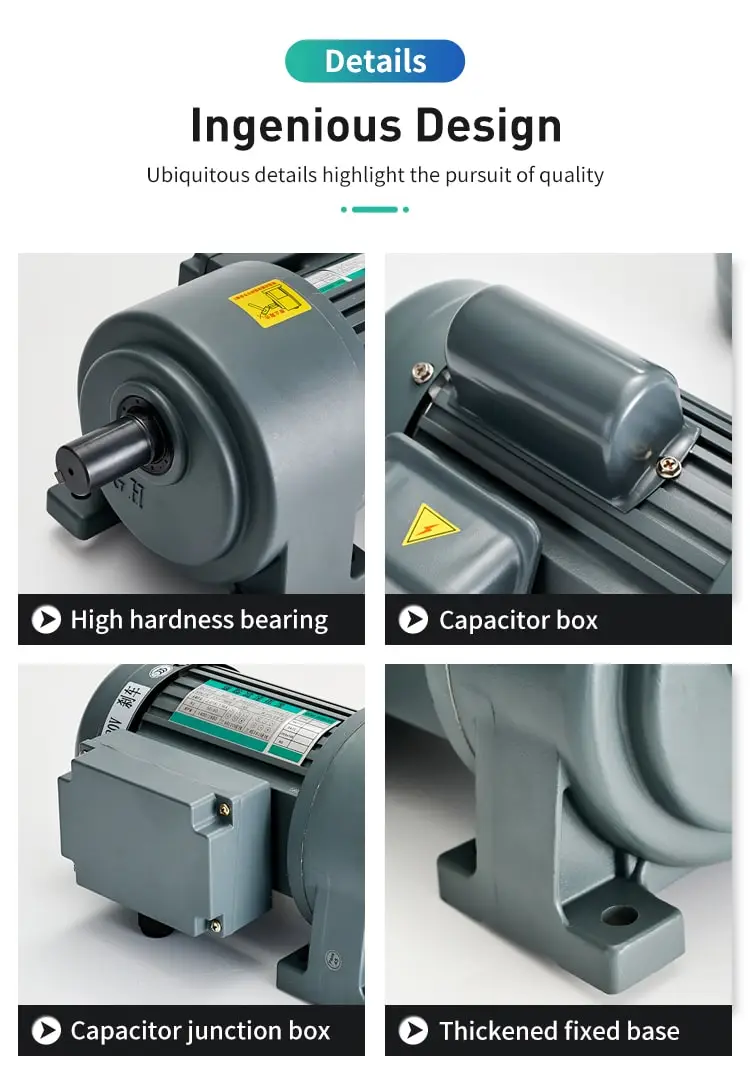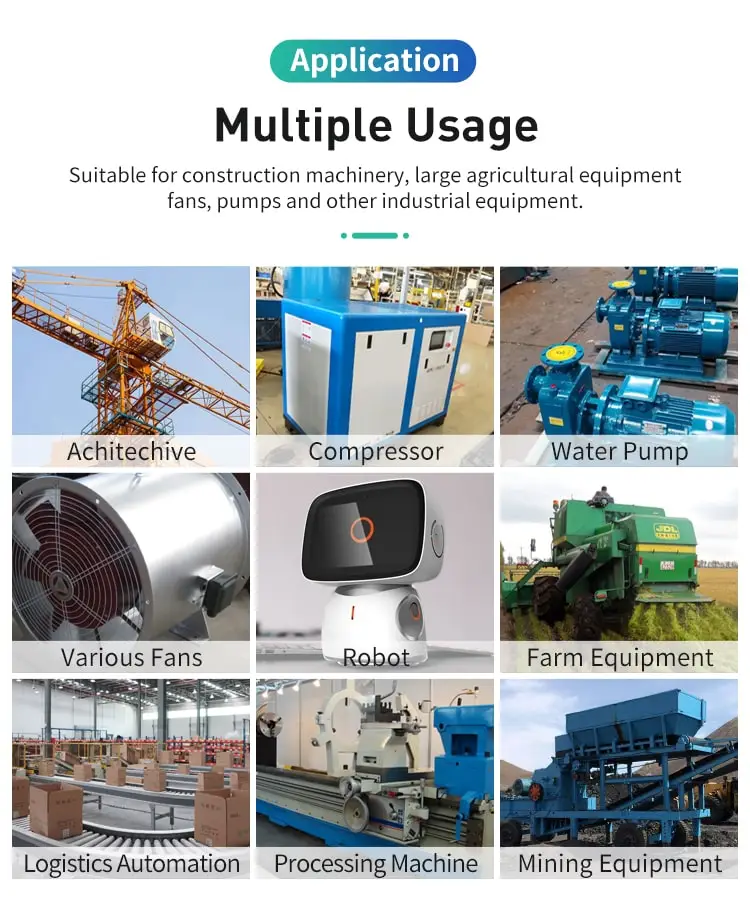By Jasmine 2023-01-16 17:18:57

#### Why squirrel cage induction motor has low starting torque?

The motor draws about 5 to 6 times the current of its full load current and produces very less torque. Most of the current is reactive in nature and does not contribute to the production of torque. That is why the starting torque of the squirrel cage indu

A squirrel cage induction motor is a type of electric motor that uses electromagnetic induction to convert electrical energy into mechanical energy. In a squirrel cage induction motor, the rotor windings are made up of a series of conductive bars that are shorted at the ends. This creates a "cage" like structure, hence the name "squirrel cage" motor.

One of the key characteristics of a squirrel cage induction motor is that it has low starting torque. In other words, it takes a relatively large amount of torque to get the rotor of a squirrel cage induction motor to start rotating. But why is this the case?

To understand why a squirrel cage induction motor has low starting torque, we need to first understand how it works. In a squirrel cage induction motor, the stator is typically made up of a series of electromagnetic coils. When an alternating current (AC) is applied to these coils, a rotating magnetic field is generated.

This rotating magnetic field then passes over the rotor windings, creating an electromagnetic field in the rotor. The interaction between the magnetic fields from the stator and rotor creates a torque, which causes the rotor to rotate and follow the direction of the rotating magnetic field.

However, when the rotor is at rest and the motor is first turned on, it takes a relatively large amount of torque to get it moving. This is because the rotor windings in a squirrel cage induction motor are shorted at the ends, which creates a high resistance. As a result, there is a high amount of electrical resistance in the rotor, which reduces the amount of torque that can be generated.

In other words, the low starting torque of a squirrel cage induction motor is due to the high resistance of the rotor windings. This high resistance makes it difficult for the rotating magnetic field from the stator to create a sufficient amount of torque to get the rotor moving.

There are a few ways to overcome the low starting torque of a squirrel cage induction motor. One common solution is to use an external resistor in the circuit to reduce the resistance of the rotor windings. This allows the rotating magnetic field from the stator to generate more torque, which makes it easier to start the motor.

Another solution is to use a different type of Induction motor that has a higher starting torque. A wound rotor induction motor, for example, has electromagnetic coils in the rotor instead of shorted conductive bars. This allows the rotor to have a lower resistance, which increases the amount of torque that can be generated and improves the starting torque of the motor.

In summary, a squirrel cage induction motor has low starting torque because the rotor windings have a high resistance. This high resistance makes it difficult for the rotating magnetic field from the stator to create enough torque to get the rotor moving. There are several solutions to this problem, including using an external resistor or switching to a different type of induction motor with a higher starting torque.